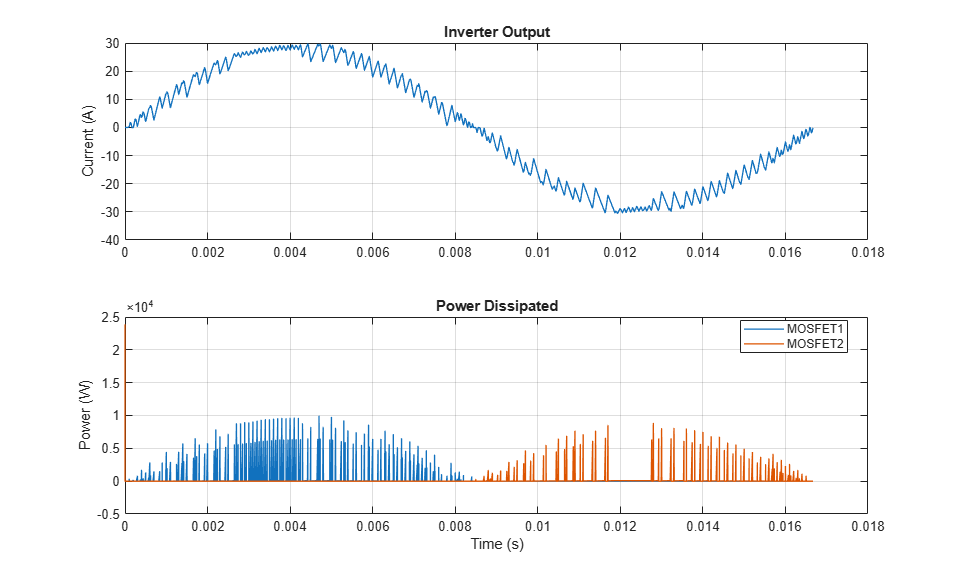# Solar Power Inverter

This example shows how to determine the efficiency of a single-stage solar inverter. The model simulates one complete AC cycle for a specified level of solar irradiance and corresponding optimal DC voltage and AC RMS current. Using the example SolarCellPowerCurveExample, the optimal values have been determined as 342V DC and 20.05A AC for an irradiance of 1000W/m^2 and panel temperature of 20 degrees Celsius. Inverter efficiency is determined in two independent ways. The first compares the ratio of AC power out to DC power in over one AC cycle. The second calculates losses by component by making use of Simscape™ logging. The small difference in calculated efficiency value is due to differences between trapezoidal integration used by the script and the greater accuracy achieved by the Simulink® variable-step solver.

### Model### Simulation Results from Simscape Logging

The plots below show the current output from the inverter and the power dissipated by two of the MOSFETs.The table below shows the power dissipated by individual components in the SolarPowerInverter model. These totals were calculated from simulation results using logged Simscape variables and the losses calculation utility ee_getPowerLossSummary.

```Efficiency = 96.7377% Losses in watts by component are as follows: LoggingNode Power _____________________________ _____ {'SolarPowerInverter.MOS2' } 18.3 {'SolarPowerInverter.MOS4' } 17.97 {'SolarPowerInverter.MOS1' } 14.07 {'SolarPowerInverter.MOS3' } 13.63 {'SolarPowerInverter.Diode4'} 3.39 {'SolarPowerInverter.Diode2'} 3.25 {'SolarPowerInverter.Diode3'} 1.7 {'SolarPowerInverter.Diode1'} 1.55 {'SolarPowerInverter.CL' } 0.4 ```